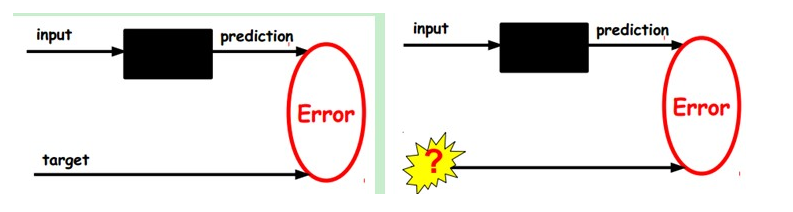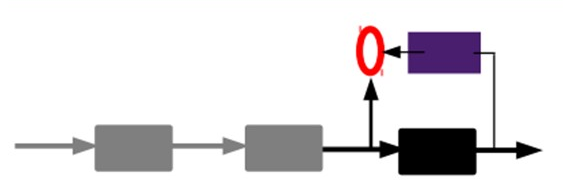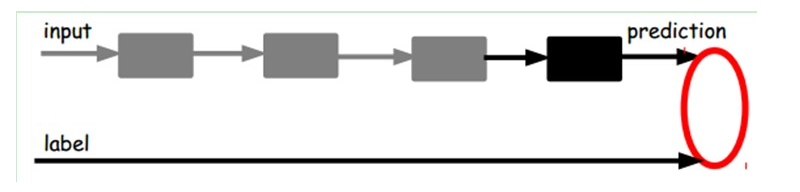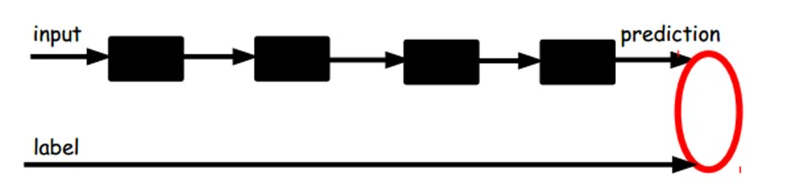# AutoEncoder自动编码器

Deep Learning最简单的一种方法是利用人工神经网络的特点，人工神经网络（ANN）本身就是具有层次结构的系统，如果给定一个神经网络，我们假设其输出与输入是相同的，然后训练调整其参数，得到每一层中的权重。自然地，我们就得到了输入I的几种不同表示（每一层代表一种表示），这些表示就是特征。自动编码器就是一种尽可能复现输入信号的神经网络。为了实现这种复现，自动编码器就必须捕捉可以代表输入数据的最重要的因素，就像PCA那样，找到可以代表原信息的主要成分。

1）给定无标签数据，用非监督学习学习特征：2）通过编码器产生特征，然后训练下一层。这样逐层训练：3）有监督微调：An autoencoder takes an input$x \in [0, 1]^d$and first maps it (with an_encoder)_to a hidden representation$x \in [0, 1]^{d^{'}}$through a deterministic mapping, e.g.:

$y=s(Wx+b)$

Where s is a non-linearity such as the sigmoid. The latent representation y , or code is then mapped back (with a_decoder)_into a reconstruction z of the same shape as x. The mapping happens through a similar transformation, e.g.:

$z=s(W^{'}x+b^{'})$

(Here, the prime symbol does not indicate matrix transposition.) z should be seen as a prediction of x , given the code y . Optionally, the weight matrix $W^{'}$ of the reverse mapping may be constrained to be the transpose of the forward mapping:$W^{'}=W^{T}$ This is referred to astied weights. The parameters of this model (namely W, b , $b^{'}$and, if one doesn’t use tied weights, also $W^{'}$) are optimized such that the average reconstruction error is minimized.

The reconstruction error can be measured in many ways, depending on the appropriate distributional assumptions on the input given the code. The traditionalsquared error$L(xz)=||x-z||^2$, can be used. If the input is interpreted as either bit vectors or vectors of bit probabilities,_cross-entropy_of the reconstruction can be used:

$L_{H}(x, z)=-\sum _{k=1}^{d} [x_klogz_k+(1-x_k)log(1-z_k)]$

The hope is that the code y is adistributed_representation that captures the coordinates along the main factors of variation in the data. This is similar to the way the projection on principal components would capture the main factors of variation in the data. Indeed, if there is one linear hidden layer (the_code)_and the mean squared error criterion is used to train the network, then the k hidden units learn to project the input in the span of the first k principal components of the data. If the hidden layer is non-linear, the auto-encoder behaves differently from PCA, with the ability to capture multi-modal aspects of the input distribution. The departure from PCA becomes even more important when we consider_stacking multiple encoders(and their corresponding decoders) when building a deep auto-encoder[Hinton06].

Because y is viewed as a lossy compression of x, it cannot be a good (small-loss) compression for all x. Optimization makes it a good compression for training examples, and hopefully for other inputs as well, but not for arbitrary inputs. That is the sense in which an auto-encoder generalizes: it gives low reconstruction error on test examples from the same distribution as the training examples, but generally high reconstruction error on samples randomly chosen from the input space.

We want to implement an auto-encoder using Theano, in the form of a class, that could be afterwards used in constructing a stacked autoencoder. The first step is to create shared variables for the parameters of the autoencoder W, b and $b^{'}$. (Since we are using tied weights in this tutorial,$W^{T}$will be used for $W^{'}$):

    def __init__(
self,
numpy_rng,
theano_rng=None,
input=None,
n_visible=784,
n_hidden=500,
W=None,
bhid=None,
bvis=None
):
"""
Initialize the dA class by specifying the number of visible units (the
dimension d of the input ), the number of hidden units ( the dimension
d' of the latent or hidden space ) and the corruption level. The
constructor also receives symbolic variables for the input, weights and
bias. Such a symbolic variables are useful when, for example the input
is the result of some computations, or when weights are shared between
the dA and an MLP layer. When dealing with SdAs this always happens,
the dA on layer 2 gets as input the output of the dA on layer 1,
and the weights of the dA are used in the second stage of training
to construct an MLP.

:type numpy_rng: numpy.random.RandomState
:param numpy_rng: number random generator used to generate weights

:type theano_rng: theano.tensor.shared_randomstreams.RandomStreams
:param theano_rng: Theano random generator; if None is given one is
generated based on a seed drawn from rng

:type input: theano.tensor.TensorType
:param input: a symbolic description of the input or None for
standalone dA

:type n_visible: int
:param n_visible: number of visible units

:type n_hidden: int
:param n_hidden:  number of hidden units

:type W: theano.tensor.TensorType
:param W: Theano variable pointing to a set of weights that should be
shared belong the dA and another architecture; if dA should
be standalone set this to None

:type bhid: theano.tensor.TensorType
:param bhid: Theano variable pointing to a set of biases values (for
hidden units) that should be shared belong dA and another
architecture; if dA should be standalone set this to None

:type bvis: theano.tensor.TensorType
:param bvis: Theano variable pointing to a set of biases values (for
visible units) that should be shared belong dA and another
architecture; if dA should be standalone set this to None

"""
self.n_visible = n_visible
self.n_hidden = n_hidden

# create a Theano random generator that gives symbolic random values
if not theano_rng:
theano_rng = RandomStreams(numpy_rng.randint(2 ** 30))

# note : W' was written as W_prime and b' as b_prime
if not W:
# W is initialized with initial_W which is uniformely sampled
# from -4*sqrt(6./(n_visible+n_hidden)) and
# 4*sqrt(6./(n_hidden+n_visible))the output of uniform if
# converted using asarray to dtype
# theano.config.floatX so that the code is runable on GPU
initial_W = numpy.asarray(
numpy_rng.uniform(
low=-4 * numpy.sqrt(6. / (n_hidden + n_visible)),
high=4 * numpy.sqrt(6. / (n_hidden + n_visible)),
size=(n_visible, n_hidden)
),
dtype=theano.config.floatX
)
W = theano.shared(value=initial_W, name='W', borrow=True)

if not bvis:
bvis = theano.shared(
value=numpy.zeros(
n_visible,
dtype=theano.config.floatX
),
borrow=True
)

if not bhid:
bhid = theano.shared(
value=numpy.zeros(
n_hidden,
dtype=theano.config.floatX
),
name='b',
borrow=True
)

self.W = W
# b corresponds to the bias of the hidden
self.b = bhid
# b_prime corresponds to the bias of the visible
self.b_prime = bvis
# tied weights, therefore W_prime is W transpose
self.W_prime = self.W.T
self.theano_rng = theano_rng
# if no input is given, generate a variable representing the input
if input is None:
# we use a matrix because we expect a minibatch of several
# examples, each example being a row
self.x = T.dmatrix(name='input')
else:
self.x = input

self.params = [self.W, self.b, self.b_prime]


Note that we pass the symbolicinputto the autoencoder as a parameter. This is so that we can concatenate layers of autoencoders to form a deep network: the symbolic output (the y above) of layer k will be the symbolic input of layer k+1

Now we can express the computation of the latent representation and of the reconstructed signal:

def get_hidden_values(self, input):
""" Computes the values of the hidden layer """
return T.nnet.sigmoid(T.dot(input, self.W) + self.b)

def get_reconstructed_input(self, hidden):
"""Computes the reconstructed input given the values of the
hidden layer
"""
return T.nnet.sigmoid(T.dot(hidden, self.W_prime) + self.b_prime)


And using these functions we can compute the cost and the updates of one stochastic gradient descent step:

    def get_cost_updates(self, corruption_level, learning_rate):
""" This function computes the cost and the updates for one trainng
step of the dA """

tilde_x = self.get_corrupted_input(self.x, corruption_level)
y = self.get_hidden_values(tilde_x)
z = self.get_reconstructed_input(y)
# note : we sum over the size of a datapoint; if we are using
#        minibatches, L will be a vector, with one entry per
#        example in minibatch
L = - T.sum(self.x * T.log(z) + (1 - self.x) * T.log(1 - z), axis=1)
# note : L is now a vector, where each element is the
#        cross-entropy cost of the reconstruction of the
#        corresponding example of the minibatch. We need to
#        compute the average of all these to get the cost of
#        the minibatch
cost = T.mean(L)

# compute the gradients of the cost of the dA with respect
# to its parameters
# generate the list of updates
(param, param - learning_rate * gparam)
for param, gparam in zip(self.params, gparams)
]



We can now define a function that applied iteratively will update the parametersW,bandb_primesuch that the reconstruction cost is approximately minimized.

    da = dA(
numpy_rng=rng,
theano_rng=theano_rng,
input=x,
n_visible=28 * 28,
n_hidden=500
)

corruption_level=0.,
learning_rate=learning_rate
)

train_da = theano.function(
[index],
cost,
givens={
x: train_set_x[index * batch_size: (index + 1) * batch_size]
}
)

start_time = timeit.default_timer()

############
# TRAINING #
############

# go through training epochs
for epoch in range(training_epochs):
# go through trainng set
c = []
for batch_index in range(n_train_batches):
c.append(train_da(batch_index))

print('Training epoch %d, cost ' % epoch, numpy.mean(c, dtype='float64'))

end_time = timeit.default_timer()

training_time = (end_time - start_time)

print(('The no corruption code for file ' +
os.path.split(__file__) +
' ran for %.2fm' % ((training_time) / 60.)), file=sys.stderr)
image = Image.fromarray(
tile_raster_images(X=da.W.get_value(borrow=True).T,
img_shape=(28, 28), tile_shape=(10, 10),
tile_spacing=(1, 1)))
image.save('filters_corruption_0.png')

# start-snippet-3
#####################################
# BUILDING THE MODEL CORRUPTION 30% #
#####################################

rng = numpy.random.RandomState(123)
theano_rng = RandomStreams(rng.randint(2 ** 30))

da = dA(
numpy_rng=rng,
theano_rng=theano_rng,
input=x,
n_visible=28 * 28,
n_hidden=500
)

corruption_level=0.3,
learning_rate=learning_rate
)

train_da = theano.function(
[index],
cost,
givens={
x: train_set_x[index * batch_size: (index + 1) * batch_size]
}
)

start_time = timeit.default_timer()

############
# TRAINING #
############

# go through training epochs
for epoch in range(training_epochs):
# go through trainng set
c = []
for batch_index in range(n_train_batches):
c.append(train_da(batch_index))

print('Training epoch %d, cost ' % epoch, numpy.mean(c, dtype='float64'))

end_time = timeit.default_timer()

training_time = (end_time - start_time)

print(('The 30% corruption code for file ' +
os.path.split(__file__) +
' ran for %.2fm' % (training_time / 60.)), file=sys.stderr)
# end-snippet-3

# start-snippet-4
image = Image.fromarray(tile_raster_images(
X=da.W.get_value(borrow=True).T,
img_shape=(28, 28), tile_shape=(10, 10),
tile_spacing=(1, 1)))
image.save('filters_corruption_30.png')
# end-snippet-4

os.chdir('../')

if __name__ == '__main__':
test_dA()


If there is no constraint besides minimizing the reconstruction error, one might expect an auto-encoder withinputs and an encoding of dimension(or greater) to learn the identity function, merely mapping an input to its copy. Such an autoencoder would not differentiate test examples (from the training distribution) from other input configurations.

Surprisingly, experiments reported in[Bengio07]suggest that, in practice, when trained with stochastic gradient descent, non-linear auto-encoders with more hidden units than inputs (called overcomplete) yield useful representations. (Here, “useful” means that a network taking the encoding as input has low classification error.)

A simple explanation is that stochastic gradient descent with early stopping is similar to an L2 regularization of the parameters. To achieve perfect reconstruction of continuous inputs, a one-hidden layer auto-encoder with non-linear hidden units (exactly like in the above code) needs very small weights in the first (encoding) layer, to bring the non-linearity of the hidden units into their linear regime, and very large weights in the second (decoding) layer. With binary inputs, very large weights are also needed to completely minimize the reconstruction error. Since the implicit or explicit regularization makes it difficult to reach large-weight solutions, the optimization algorithm finds encodings which only work well for examples similar to those in the training set, which is what we want. It means that the_representation is exploiting statistical regularities present in the training set,_rather than merely learning to replicate the input.

There are other ways by which an auto-encoder with more hidden units than inputs could be prevented from learning the identity function, capturing something useful about the input in its hidden representation. One is the addition ofsparsity(forcing many of the hidden units to be zero or near-zero). Sparsity has been exploited very successfully by many[Ranzato07][Lee08]. Another is to add randomness in the transformation from input to reconstruction. This technique is used in Restricted Boltzmann Machines (discussed later inRestricted Boltzmann Machines (RBM)), as well as in Denoising Auto-Encoders, discussed below.